Download PresentationLaplace Transform

# Laplace Transform - PowerPoint PPT Presentation

Laplace Transform. BIOE 4200 . Why use Laplace Transforms?. Find solution to differential equation using algebra Relationship to Fourier Transform allows easy way to characterize systems No need for convolution of input and differential equation solutionI am the owner, or an agent authorized to act on behalf of the owner, of the copyrighted work described.
Download Presentation## Laplace Transform

An Image/Link below is provided (as is) to download presentation

Download Policy: Content on the Website is provided to you AS IS for your information and personal use and may not be sold / licensed / shared on other websites without getting consent from its author.While downloading, if for some reason you are not able to download a presentation, the publisher may have deleted the file from their server.

- - - - - - - - - - - - - - - - - - - - - - - - - - E N D - - - - - - - - - - - - - - - - - - - - - - - - - -
Presentation Transcript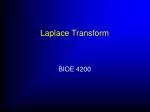### Laplace Transform

BIOE 4200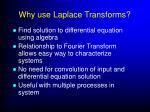Why use Laplace Transforms?
• Find solution to differential equation using algebra
• Relationship to Fourier Transform allows easy way to characterize systems
• No need for convolution of input and differential equation solution
• Useful with multiple processes in system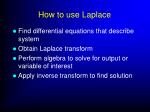How to use Laplace
• Find differential equations that describe system
• Obtain Laplace transform
• Perform algebra to solve for output or variable of interest
• Apply inverse transform to find solution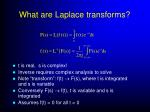What are Laplace transforms?
• t is real, s is complex!
• Inverse requires complex analysis to solve
• Note “transform”: f(t)  F(s), where t is integrated and s is variable
• Conversely F(s)  f(t), t is variable and s is integrated
• Assumes f(t) = 0 for all t < 0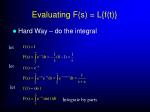Evaluating F(s) = L{f(t)}
• Hard Way – do the integral

let

let

let

Integrate by parts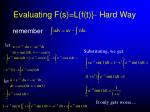Evaluating F(s)=L{f(t)}- Hard Way

remember

let

Substituting, we get:

let

It only gets worse…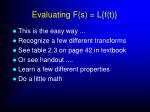Evaluating F(s) = L{f(t)}
• This is the easy way ...
• Recognize a few different transforms
• See table 2.3 on page 42 in textbook
• Or see handout ....
• Learn a few different properties
• Do a little math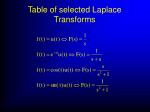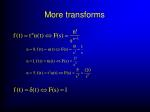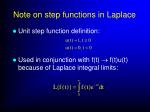Note on step functions in Laplace
• Unit step function definition:
• Used in conjunction with f(t)  f(t)u(t) because of Laplace integral limits:Properties of Laplace Transforms
• Linearity
• Scaling in time
• Time shift
• “frequency” or s-plane shift
• Multiplication by tn
• Integration
• DifferentiationProperties: Linearity

Example :

Proof :Properties: Scaling in Time

Example :

Proof :

letProperties: Time Shift

Example :

Proof :

letThe “D” Operator
• Differentiation shorthand
• Integration shorthand

if

if

then

thenProperties: Integrals

Proof :

Example :

let

If t=0, g(t)=0

for

so

slower thanDifference in
• The values are only different if f(t) is not continuous @ t=0
• Example of discontinuous function: u(t)Properties: Nth order derivatives

let

NOTE: to take

you need the value @ t=0 for

called initial conditions!

We will use this to solve differential equations!Properties: Nth order derivatives

Now apply again

let

then

remember

Can repeat forRelevant Book Sections
• Modeling - 2.2
• Linear Systems - 2.3, page 38 only
• Laplace - 2.4
• Transfer functions – 2.5 thru ex 2.4